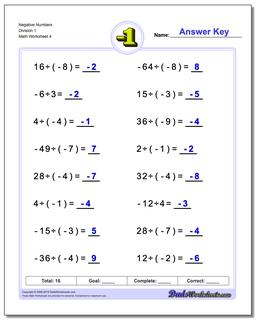One Dad. Four daughters. 9,268 worksheets... and counting!# Negative Numbers Division 1 (Fourth Worksheet)

PropertyValue
DescriptionNegative Numbers Division 1: The worksheets in this section introduce negative numbers integers in multiplication and division math problems. All problems deal with smaller integers that can be solved without multi-digit multiplication or long division. (Fourth Worksheet)
Resource TypeWorksheet